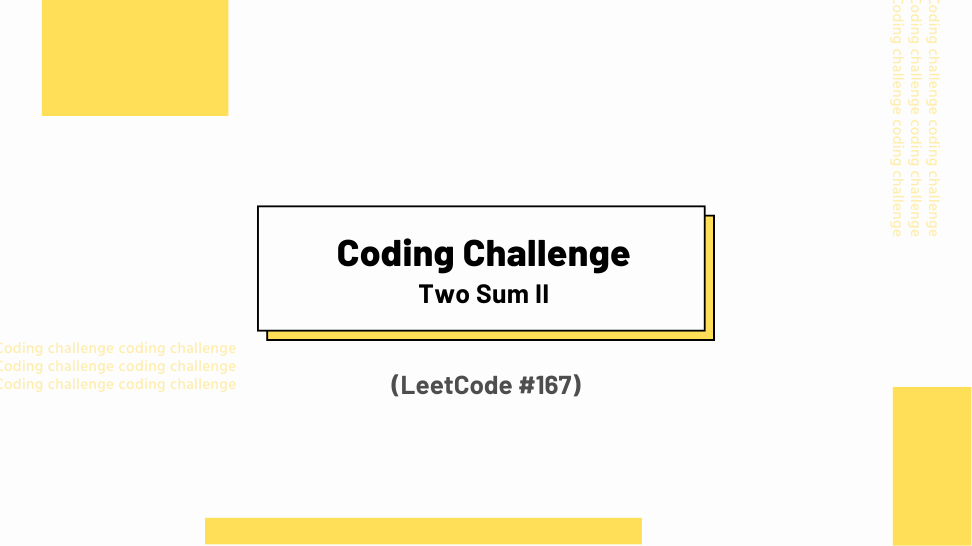## Challenge Statement

• Given a 1-indexed array of integers numbers that is already sorted in non-decreasing order, find two numbers such that they add up to a specific target number. Let these two numbers be numbers[index1] and numbers[index2] where 1 <= index1 < index2 <= len(numbers).
• Return the indices of the two numbers, index1 and index2, added by one as an integer array [index1, index2] of length 2.
• The tests are generated such that there is exactly one solution. You may not use the same element twice.
• Your solution must use only constant extra space.
• This challenge corresponds to LeetCode #167.

### Constraints

• `2 <= len(numbers) <= 3 * 104`
• `-1000 <= numbers[i] <= 1000`
• numbers is sorted in non-decreasing order.
• `-1000 <= target <= 1000`
• The tests are generated such that there is exactly one solution.
• s consists only of printable ASCII characters.

### Example 1:

Input: `numbers = [2, 7, 11, 15]`, `target = 9`

Output: `[1, 2]`

Explanation: The sum of 2 and 7 is 9. Therefore, index1 = 1, index2 = 2. We return [1, 2].

### Example 2:

Input: `numbers = [2, 3, 4]`, `target = 6`

Output: `[1, 3]`

Explanation: The sum of 2 and 4 is 6. Therefore index1 = 1, index2 = 3. We return [1, 3].

### Example 3:

Input:` numbers = [-1, 0]`, `target = -1`

Output: `[1, 2]`

Explanation: The sum of -1 and 0 is -1. Therefore index1 = 1, index2 = 2. We return [1, 2].

## Solution

Below is my solution and some test cases. This solution has a linear time complexity O(n) and a constant space complexity O(1), where n is the length of the input list.